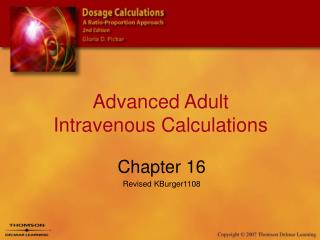DownloadDownload PresentationTélécharger la présentation- - - - - - - - - - - - - - - - - - - - - - - - - - - E N D - - - - - - - - - - - - - - - - - - - - - - - - - - -
##### Presentation Transcript

2. IV Heparin • Anticoagulant – prevention of clot formation • High alert medication • All heparin orders, dosage, vial, amount and calculations should be checked by another nurse • Available in various dosage strengths • See figure 16-1 page 406 in Pickar

3. Heparin Flow Rate • Set up a ratio-proportion with:- supply dosage strength [units per ml]- ordered infusion dose [units/hr per units/ml] • Example: Order: 500 mL D5W with Heparin 25,000 units to infuse @ 850 units / hr25,000units = 850units/hr 500 mL X mL/hr 25,000X = 425,00025,000 25,000 = 17 mL/hr

4. Heparin Flow RatesTry these… 1. The doctor orders heparin to infuse at 800 unit per hour. The pre-mixed intravenous solution available is 250mL D5W with 25000 units heparin added. Calculate the infusion rate for delivery with an infusion pump. 2. The doctor orders heparin to infuse at 750 unit per hour. The pre-mixed intravenous solution available is 500mL D5W with 25000 units heparin added. Calculate the infusion rate for delivery with an infusion pump. 8 mL / hr 15 mL / hr

5. See p.408 Pickar Standard Weight-Based Heparin Protocol 1. Weight in kilograms. Required for order to be processed ______kg 2. Heparin 25,000 units in 250mL 1/2NS. Bolus dosage strength 1000units/mL. • APTT q6hrs or 6 hrs after rate change; daily for 2 consecutive therapeutic APTTs. • CBC initially and repeat every ______day[s] • Obtain APTT and PT/INR on day one prior to initiation of therapy • Guaiac stool initially then every _____day[s] until heparin d/c • Neuro checks every _____hrs while on heparin • Discontinue APTT and CBC once heparin is discontinued • Notify physician of any bleeding problems • Bolus with 80 units/kg. Start drip at 18 units/kg/hr • If APTT is less than 35 seconds Rebolus with 80units/kg and increase rate by 4 units/kg/hr • If APTT is 36-44 seconds Rebolus with 40units/kg and increase rate by 2 units/kg/hr • If APTT is 45-75 seconds Continue current rate • If APTT is 76-90 seconds Decrease rate by 2 units/kg/hr • If APTT is greater than 90 seconds Hold heparin for 1 hour and decrease rate by 3 units/kg/hr

6. Using the Heparin Protocol • Patient weight = 110 lbsConvert to kilograms = 50 kg • Calculate the heparin bolusProtocol states: Bolus with 80 units/kg80 units X 50 kg = 4000 units • Calculate the bolus in millilitersProtocol states: Bolus dosage strength 1000 units/mL1000 Units = 4000 Units 1 mL X mL = 4 mL

7. Using the Heparin Protocol • Calculate the starting infusion rateProtocol states: Start drip at 18 units/kg/hrMultiply by pt weight of 50 kg: 18units X 50 kg = 900units/hrProtocol states: Heparin 25,000 Units in 250 mL of ½ NS.25000 Units = 900 Units/hr250 mL X mL/hrX = 9 mL/hr • Adjust infusion rate according to APTT results. Follow protocol guidelines.Examples: Client has APTT of: 407088 Rebolus with 40units/kg/hr & increase rate by 2 units/kg/hr Continue current rate Decrease rate by 2units/kg/hr

8. IV Flow Rate Ordered “Per Minute” • Critical Care IV calculations • Determine electronic IV infusion rate (mL per hour) for IV medications ordered per minute (such as mg per min) • Step 1: Calculate dosage in mL per min • Step 2: Calculate flow rate in mL per hour

9. 2000 mg EXAMPLE: Ordered lidocaine 2g IV in 500 mL D5W @ 2 mg/min Step 1Calculate ml/min:2000mg = 2mg/min500mL X mL/min X = 0.5mL/min Step 2Calculate ml/hr:0.5mL = X mL/hr 1 min 60 min/hrX = 30ml//hr

10. IV Flow Rate Ordered by Kilogram per minute • Calculate electronic IV infusion rate (mL per hour) for IV medications ordered by weight per minute (such as mg per kg per min): Step 1- Calculate desired dosage per minute Step 2- Calculate desired dosage in mL per minute Step 3- Calculate flow rate in mL per hour

11. EXAMPLE:250mL of D5W with 225 mg of dobutamine to infuse @ 3mcg/kg/min.Client weighs 50 kg 0.003 mg Step 1- Calculate desired dosage per minute0.003mg X 50 kg = 0.15mg/minute Step 2- Calculate desired dosage in mL per minute225mg = 0.15mg/min250mL X mL/min X = 0.17 ml/min Step 3- Calculate flow rate in mL per hour0.17mL = X mL/hr1 min 60min/hr X = 10ml/hr

12. Titrating IV Drugs • Mostly seen in Critical care and/or OB • Started with bolus • Then increased to produce desired effect • Started at lowest dosage and increased or decreased as needed • Examples:Dopamine, Pitocin

13. Example: RL 1000mL with Magnesium Sulfate 20g. Start with bolus of 4g for 30 min, then maintain continuous infusion @ 2g/hr. Step 1:Calculate bolus in g/mL:20g = 4g1000mL X mL X = 200mLshould infuse over 30 min Step 2:Calculate bolus in mL/hr200mL = XmL/hr 30 min 60 min/hrX = 400mL/hr Step 3:Calculate continuous infusion rate by repeating steps 1 and 2 using 2 g instead of 4g.